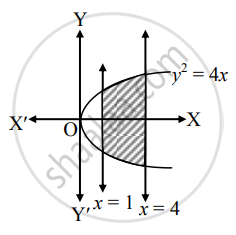# Choose the correct alternative : The area of the region bounded by y2 = 4x, the X-axis and the lines x = 1 and x = 4 is _______. - Mathematics and Statistics

MCQ

Choose the correct alternative :

The area of the region bounded by y2 = 4x, the X-axis and the lines x = 1 and x = 4 is _______.

#### Options

• 28 sq. units

• 3 sq. units

• (56)/(3)"sq. units"

• (63)/(7)"sq. units"

#### SolutionRequired area = 2int_1^4y*dx

= 2int_1^4 2sqrt(x)*dx

= 4[x^(3/2)/(3/2)]_1^4

= (8)/(3)[(4)^(3/2) - (1)^(3/2)]

= (8)/(3)(8 - 1)

= (56)/(3)"sq.units".

Is there an error in this question or solution?
Chapter 7: Applications of Definite Integration - Miscellaneous Exercise 7 [Page 157]

#### APPEARS IN

Balbharati Mathematics and Statistics 1 (Commerce) 12th Standard HSC Maharashtra State Board
Chapter 7 Applications of Definite Integration
Miscellaneous Exercise 7 | Q 1.2 | Page 157
Share